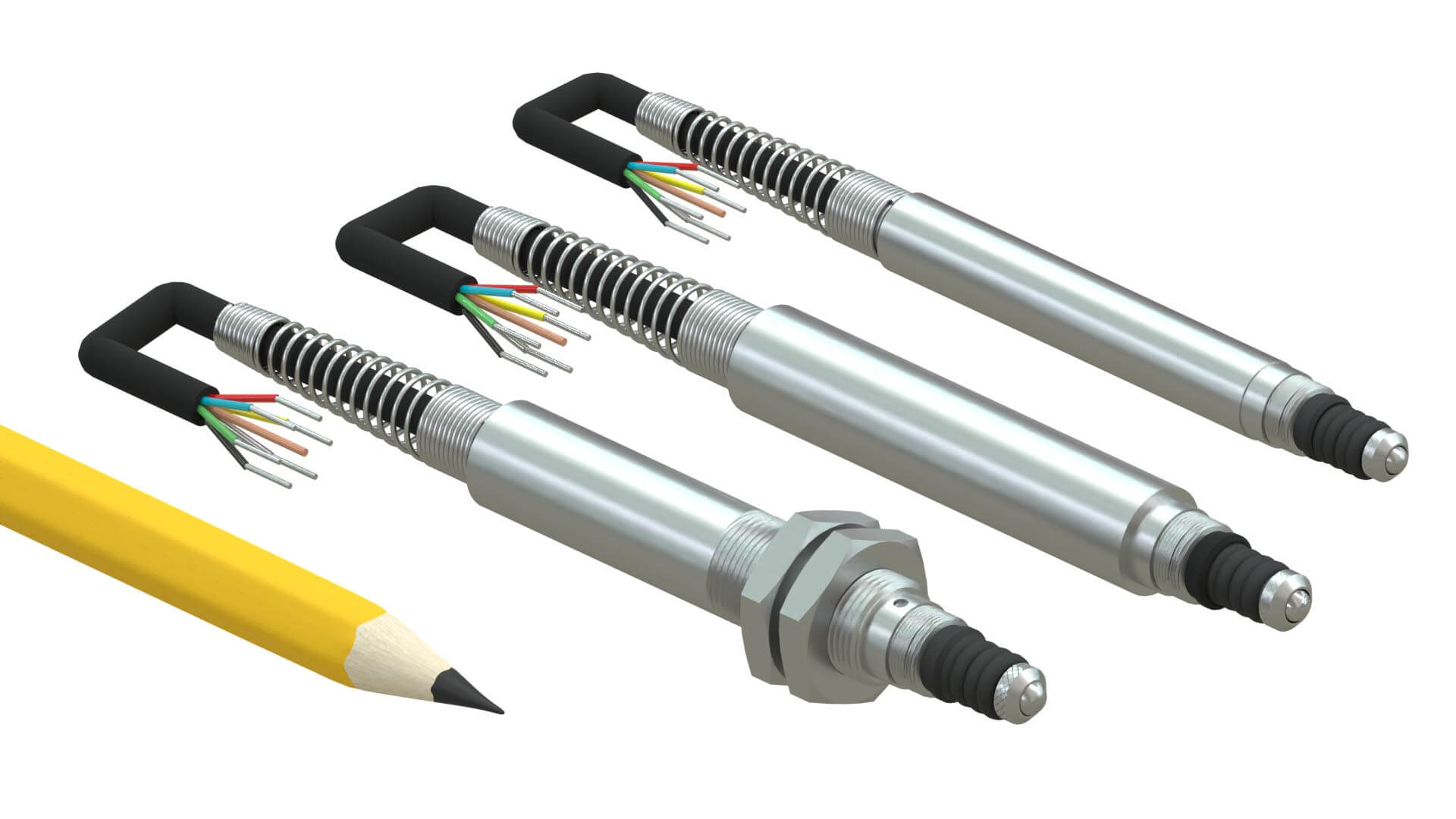# Techniques for Measuring the Primary Impedance of an LVDT

Periodically the need arises to be able to measure the primary impedance of an LVDT to ensure that it will work with a particular version of signal conditioning electronics. Typical LVDT signal conditioner systems are built to work with a primary impedance of 200 Ohms or higher, but some systems cannot supply enough excitation power to operate an ordinary industrial LVDT. If a user is uncertain of the primary impedance of a particular LVDT, it may be necessary for that user to actually measure it.

However, before explaining how to do it, it is important to be certain that the user understands that one cannot measure impedance using the resistance ranges of a multimeter, even though they are both measured in Ohms. Those ranges of a multimeter indicate DC resistance (DCR).

Impedance is an AC phenomenon, which is a function of and determined by frequency. There are many inexpensive hand-held instruments that can measure R-L-C circuit element parameters which can then be used to calculate inpedance. However, these devices are often unable to make measurements at the desired frequency. There are also expensive instruments called Impedance Meters that can directly measure the impedance of an R-L-C circuit at some particular frequency. These meters usually have a variable frequency source or some selection of common reference frequencies available to provide an impedance reading. Finally, Impedance Bridges are older laboratory devices to measure impedance by measuring the R-L-C circuit element parameters which can then be used to calculate inpedance

In the absence of direct reading instruments to measure impedance, there is a very fundamental method of measuring the scalar (magnitude) component of the primary impedance of any LVDT on the laboratory bench by using Ohm's Law for AC circuits, as described in the steps below.

1. Make sure the core of the LVDT is approximately at its Null position inside the LVDT's bore.
2. Hook up an audio frequency sine wave oscillator to the LVDT's primary leads or connector, being sure both of the LVDT's secondaries are not connected to anything.
3. Set the oscillator at or near the LVDT's nominal excitation frequency, typically 2.5 or 3.0 kHz.
4. Use a precision true-RMS AC voltmeter with enough bandwidth at the above frequency to measure the voltage being applied to the LVDT's primary at its nominal AC input, typically 3 V.
5. Use a precision true-RMS AC milliameter, again with enough bandwidth at the frequency above, connected in series with the LVDT's primary to measure the AC current being drawn.
6. Use a calculator to divide the RMS voltage from step 4 by the RMS current in step 5. If the AC current is in milliamperes and the AC excitation is in Volts, using Ohm's Law for AC circuits, the quotient is the magnitude in kilohms of the primary impedance at the excitation frequency.
7. To know the LVDT's input power in milliwatts, multiply AC voltage (V) by AC current (mA).
8. For an LVDT that is already connected to an LVDT signal conditioner and being powered at or near the LVDT's nominal excitation frequency, it is only necessary to follow steps 1, 4, 5, and 6 above to gather the parameters needed to calculate the LVDT's primary impedance.